# Combined Spatial-Spectral Hyperspectral Image Classification Based on Adaptive Guided Filtering

Combined Spatial-Spectral Hyperspectral Image Classification Based on Adaptive Guided Filtering

Liang Huang Shenkai Nong Xiaofeng Wang Xiaohang Zhao Chaoran Wen Ting Nie

Changchun Institute of Optics, Fine Mechanics and Physics, Chinese Academy of Sciences, Changchun 130033, China

University of Chinese Academy of Sciences, Beijing 100049, China

Corresponding Author Email:
nieting@ciomp.ac.cn
Page:
745-754
|
DOI:
https://doi.org/10.18280/ts.390240
28 November 2021
|
Accepted:
17 January 2022
|
Published:
30 April 2022
| Citation

OPEN ACCESS

Abstract:

Hyperspectral image classification has a low accuracy in the face of a small training set. To solve the problem, this paper proposes a combined spatial-spectral hyperspectral image classification approach based on adaptive guided filtering. From coarse to fine classification, the local binary pattern (LBP) histogram features were improved, the spatial contrast description was enhanced, and enhanced spatial-spectral features were prepared through Gabor transform of different scales and directions, combined with super pixel blocks. Then, the pre-classification was completed by the support vector machine (SVM) classifier. To reduce noise interference, the pre-classification results were filtered again by a guided filter based on the adaptive regularization factor. To verify its effectiveness, the proposed approach was compared with the state-of-the-arts approaches through repeated experiments. The comparison shows that our approach achieved a high classification accuracy, while suppressing noise interference. This research provides a new tool for hyperspectral image classification with a small training set.

Keywords:

combined spatial-spectral hyperspectral image classification, enhanced spatial-spectral information, improved description of local binary pattern (LBP), adaptive guided filtering

1. Introduction

The hyperspectral remote sensing images contain hundreds of continuous bands, which provide a huge amount of spectral and spatial information. Despite this advantage, there are several defects with such images: the adjacent continuous bands are often redundant and overlapped; the massive amount of data makes it difficult to store or transmit on aircrafts or satellites; the images are too complex to be processed easily.

Judging by the utilization of spatial information, the classification algorithms of hyperspectral remote sensing images can be divided into two categories: spectral information-based algorithms, and spatial-spectral information-based algorithms. The Gaussian maximum likelihood classifier is a classic spectral information-based algorithm for hyperspectral remote sensing images. However, the classifier is rather slow, due to the high dimensions of the spectrum in such images. To solve the problem, Vapnik et al.  proposed the support vector machine (SVM), which works well on small samples. The SVM has been widely applied to classify hyperspectral remote sensing images.

The automatic extended attribute profiles (AEAP)  is an algorithm based on automatic extended attributes. Low-rank representations  and wavelet filters  are typical methods based on spatial features. The Markov model  is the first to adopt spatial feature extraction. By the Markov model, the spatial and contextual information are integrated into the classification framework, and successfully applied to classify hyperspectral images. Pesaresi and Benediktsson  introduced the opening and closing series of the reconstruction operator, and utilized the predefined and scale increasing structural element (SE) to construct the morphological profile (MP) of the original image. Myint et al. analyzed the texture of remote sensing images through wavelet transform, and demonstrated the effectiveness of the method .

The traditional remote sensing image classification algorithms only process the spatial information, failing to consider the spectral features or fully utilize the spectral information of the images. There is ample room to improve the performance of these algorithms. In fact, the traditional algorithms cannot be applied to process hyperspectral remote sensing images. Otherwise, different classes of objects may be attributed to the same spectrum, or the same class of objects may be assigned to different spectra; some noises would be generated during the processing; small pixels are often classified incorrectly.

With the increase of spatial resolution, the hyperspectral images will witness a growth in within-class dispersion, and a decline in between-class dispersion, making the spectral information less discriminable. Therefore, many scholars started to mien the spatial information of hyperspectral images. Through image decomposition, Li et al.  divided the original image into a base layer containing largescale intensity change, and a detail layer that captures small details, and proposed a weighted average method based on guided filtering to fuse the two layers, using spatial consistency. Kang et al.  put forward an edge-preserving filter (EPF) for combined spatial-spectral hue-saturation-intensity (HSI) classification. The EPF classifies the original image with the SVM, sets up the initial probability map, and corrects the map with bilateral or guided filter, thereby enhancing the final classification accuracy. Liu et al.  presented an unsupervised dimensionality reduction method called super-pixelwise collaborative-representation graph embedding (SPCRGE). Kang et al.  developed the image fusion and recursive filter (IFRF) for HSI feature extraction. The IFRF splits the HSI samples into multiple subsets, according to the adjacent hyperspectral bands, fuses each subset by the average method, extracts the features from each fused subset with the recursive filter, and finally adopts the SVM for classification. To improve classification accuracy, Li et al.  designed a reduced dimensional convolutional neural network (CNN) with a two-dimensional (2D) Gabor filter based on local similarity projection (LSP). Bhatti et al.  introduced a novel algorithm to classify hyperspectral images: the SVM with shape-adaptive reconstruction and smoothed total variation (SaR-SVM-STV).

In recent years, deep learning has performed excellently on hyperspectral image classification. Compared with traditional supervised methods, deep learning can automatically learn data features, and classify the original data. As a result, deep learning is applicable to various tasks and scenes. The CNN, a typical model of deep learning, piques the interests of many hyperspectral image researchers, owing to its powerful ability of feature extraction [14-18]. For example, Haut et al. proposed a data enhancement strategy with random occlusions, and devised a 2D CNN for hyperspectral image classification based on spatial features .

Facing the lack of labeled HSI samples, many researchers resort to graph-based methods to realize semi-supervised classification of hyperspectral images . Hong et al.  integrated the CNN and graph convolutional network (GCN) through addition, element-by-element multiplication, and serial connection, and tested the performance gain of the integrated approach. Ma et al.  proposed the multiscale random convolution broad learning system (MRC-BLS). With the spatial feature learning of adaptive weighted mean filter as the convolutional kernel, the MRC-BLS extracts local spatial features on the first layer, imports the multiscale feature maps obtained by random kernels of various sizes to the width learning classifier, and thus achieves a high performance in classifying HSI images. Zhou et al.  proposed a collaborative encoding model that perceives spatial peak information.

To achieve a high classification accuracy, most deep learning methods need lots of labeled samples. The numerous layers and multiple parameters add to the time and labor costs of network training, and require high hardware configurations. Despite their high accuracy, deep networks alone cannot classify hyperspectral images rapidly and cost effectively.

Hyperspectral image classification has a low accuracy in the face of a small training set. To solve the problem, this paper proposes a combined spatial-spectral hyperspectral image classification approach based on adaptive guided filtering. From coarse to fine classification, a combined spatial-spectral classification framework was established through the fusion of multiple features. The main contributions are as follows:

(1) The local binary pattern (LBP) descriptor was improved, the spatial information description was enhanced, and the spatial-spectral information was reinforced, thus increasing the classification accuracy. A guided filter was developed based on the adaptive regularization factor, and applied to filter the initial classification results of the SVM again. The secondary filtering improves classification accuracy, and reduces noise interference.

(2) The proposed method, which combines Gabor transform  and the spatial information of super pixel blocks, can achieve a high classification accuracy on small samples.

The local binary pattern (LBP) histogram features were improved, the spatial contrast description was enhanced, and enhanced spatial-spectral features were prepared through Gabor transform of different scales and directions, combined with super pixel blocks. Then, the pre-classification was completed by the support vector machine (SVM) classifier. To reduce noise interference, the pre-classification results were filtered again by a guided filter based on the adaptive regularization factor. To verify its effectiveness, the proposed approach was compared with the state-of-the-arts approaches through repeated experiments. The comparison shows that our approach achieved a high classification accuracy, while suppressing noise interference. This research provides a new tool for hyperspectral image classification with a small training set.

2. Methodology

This paper proposes a coarse-to-fine classification method, which contains four steps: dimensionality reduction through principal component analysis (PCA) ; preprocessing of basic spatial-spectral data; initial classification by the SVM; secondary optimization of classification results by adaptive guided filter. Figure 1 shows the overall flow of our approach.

## 1.png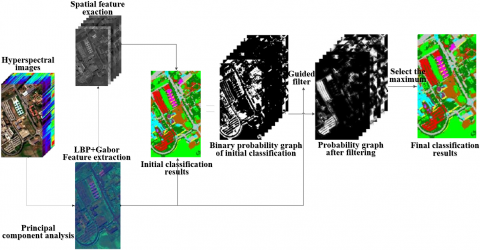Figure 1. Flow chart of spatial-spectral fusion hyperspectral classification based on guided filtering

2.1 PCA dimensionality reduction

Table 1. Principal components of hyperspectral images

 Principal component Indian Pines University of Pavia 1 68.97% 56.78% 2 24.19% 35.06% 3 1.85% 4.49% 4 0.87% 0.35% 5 0.74% 0.26% 6 0.57% 0.23% 7 0.45% 0.17% 8 0.41% 0.12% 9 0.36% 0.10% 10 0.34% 0.08% Sum of top 10 principal components 98.75% 97.64%

Owing to the sheer volume of hyperspectral images, it is very slow to directly classify these images. The classification accuracy may be suppressed by the noises that appear during the classification process. To improve efficiency and reduce noise interference, this paper processes the principal components of the spectrum through the PCA. Specifically, the PCA was adopted to extract the principal components of two common datasets: Indian Pines and University of Pavia. The statistics are shown in Table 1. It can be seen that most scene information can be preserved by extracting the spatial features of the top three principal components of the two scenes.

2.2 Spatial-spectral data processing

Our spatial-spectral data processing method combines spatial information with spectral information. There are three major steps of our method: Firstly, the Gabor filter is adopted to extract the texture information of images of different scales and directions. Next, the LBP histogram operator is improved to illustrate the spatial contrast of images. Finally, each pixel is subjected to super pixel operation, and the image information is better depicted with three different types of features.

The Gabor filter can effectively extract the spatial features of ground objects, and enhance the classification accuracy of combined spatial-spectral information. In essence, the Gabor filter iteratively substitutes the pixel values after convolution. The filtering effect depends on the setting of the kernel function. The Gabor filter can be expressed as:

$g(x, y ; \lambda, \theta, \psi, \sigma, \gamma)=\exp \left(-\frac{x^{\prime 2}+\gamma^{2} y^{\prime 2}}{2 \sigma^{2}}\right) * \exp \left(i\left(2 \pi \frac{x}{\alpha}+\psi\right)\right)$        (1)

where, x and y are the coordinates of a pixel; $\lambda$ is the wavelength, which is generally measured by the number of pixels, and no longer than 1/5 of the input image size; $\theta$=0-$2 \pi$ is the stripe direction; $\psi$=0 is the phase shift; $\gamma$=0.5 is the spatial aspect ratio; $\sigma$ is the Gaussian factor standard deviation of the Gabor function (its value is related to the previous parameters). The parameter values of the Gabor kernel function need to be adjusted, according to the classification needs. Different parameters lead to different spatial information.

Apart from that, this paper proposes an adaptive LBP histogram feature descriptor for hyperspectral data. The LBP operator has been widely applied to texture feature analysis . The thresholding of the LBP feature of the central pixel is implemented by comparing the gray value of each pixel with that of any other adjacent pixel. Then, the central pixel is encoded with a certain weight. The LBP operation can be expressed as:

$\mathrm{LBP}_{\mathrm{k}, \mathrm{r}}\left(x_{c}\right)=\sum_{p=0}^{k-1} s\left(x_{\mathrm{p}}-x_{c}\right) 2^{p}$       (2)

where, xp and xare the gray values of the neighborhood and the central pixel, respectively; k is the number of pixels in the neighborhood with xc as the center, and r as the radius.

The original LBP only considers the relationship between the gray values of the central pixel and the adjacent pixels, without taking account of the contrast relationship. The original LBP is illustrated in Figure 2. In Figure 2(a), the central pixel has a very small contrast with the adjacent pixels. In Figure 2(a), that contrast is very high. The two pixel distributions differ significantly in contrast. But their original LBP values are the same. This is clearly unfavorable for image classification.

## 2.1.png(a) LBP: 00001111

## 2.2.png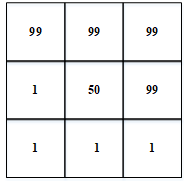(b) LBP: 00001111

Figure 2. Original LBP

In response to the above problem, this paper proposes the modified LBP operator (MLBP), which automatically adapts to the contrast changes. The local contrast mapping between xp and xc is computed to find the maximum (maxC) and minimum (minC) of the contrast mapping. Then, the value range of maxC and minC is divided into L levels. Then, each contrast corresponds to a level. The MLBP operation can be expressed as:

$l_{p}=\left[\frac{\left(g_{p}-g_{c}\right)-\min C}{(\max C-\min C) / L\quad}\right]$       (3)

where, maxC and minC are the maximum and minimum contrasts between the central pixel and its neighborhood, respectively; L is the number of layers (if the result is greater than L, then lp=L); gp and gc are the gray values of the neighborhood and the central pixel, respectively.

Accordingly, the binary descriptor $S_{p}$ of the contrast adaptive MLBP can be expressed as:

$S_{p}=\left\{\begin{array}{l}1, l_{p}=L \\ 0, l_{p} \neq i\end{array}\right.$       (4)

To obtain rotation invariance, the binary descriptor is illustrated with the number of switches between zero and one. Finally, the rotation-invariant MLBP can be expressed as:

$M L B P_{P, R}^{i}=\left\{\begin{array}{c}\sum_{p=0}^{P-1} S_{p}, \text { if }(U(M L B P)<=2) \\ P+1\end{array}\right.$       (5)

where, $U(M \mathrm{LBP})$ is the number of switches between zero and one in the n-digit binary data.

Figure 3 gives an example of the MLBP description process, where zero and one are switched for four times.

## 3.png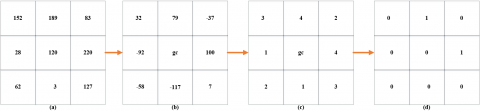Figure 3. An example of MLBP description: (a) Original image, (b) Contrast diagram, (c) Contrast level calculation diagram, (d) Initial binary description 00000101

## 4.1.png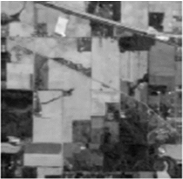(a) The first principal component

## 4.2.png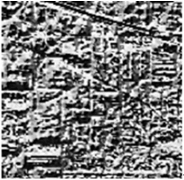(b) MLBP coding of the Indian Pines dataset

Figure 4. The example of MBLP coding

## 5.png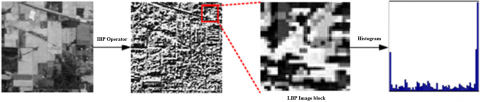Figure 5. The example of MBLP feature extraction

During MLBP description, a pixel can be selected as the center of a square or circle, and the value of another pixel in that area can be estimated through bilinear interpolation. By our approach, the binary descriptions of Figures 3(a) and 3(b) are 11101111 and 00001111, respectively, and the corresponding rotation-invariant MLBPs are 1 and 2, respectively. Obviously, the MLBP varies with the contrast distributions.

Figure 4 presents the MLBP spatial features of Indian Pines. The first principal component obtained by PCA is given in Figure 4(a), and the texture description of the entire image obtained by the MLBP is displayed in Figure 4(b).

Taking the MLBP histogram statistics as a spatial feature, the MLBP code of each pixel is solved by formula (5). Then, the MLBP histogram of the pixels is obtained through histogram statistics. After that, the frequency of occurrence of each MLBP is treated as a spatial feature. Figure 5 explains the MLBP feature extraction.

To utilize neighborhood information, each pixel in the original image is subjected to patch operation. The 5×5 area centering on the pixel is composed into a super pixel block. These super pixel blocks form a new dataset. In the new dataset, each sample contains the neighborhood information of the original sample, thereby enhancing the spectral features. The specific algorithm is as follows:

The original dataset is assumed as:

$(\mathrm{x}, \mathrm{y}), \mathrm{x} \in R^{n}$        (6)

The original spectral data go through PCA feature extraction, with x being the dimensionality of image spectrum. With n =200, and k =22, the dimensionally reduced sample data can be expressed as:

$\left(x_{1}, y\right), x_{1} \in R^{k}$       (7)

where, x1 is the spectral dimensionality of the dimensionally reduced image. Each pixel goes through the patch operation to obtain a super pixel block. If the blocks exceed the image boundaries, the extra part is filled with the data of the current pixels. In this way, a new dataset is obtained with super pixel blocks as samples. The patch size is set to 5. In the new dataset, each sample corresponds to:

$\left( {{x}_{2}},y \right),{{x}_{2}}\in {{R}^{\text{patc}{{\text{h}}_{\text{s}}}\text{ize}\times \text{patc}{{\text{h}}_{\text{s}}}\text{ize}\times \text{k}}}$       (8)

Then, $x_{2}$ can be expended as a one-dimensional data along the third dimension:

$\left( {{x}_{3}},y \right),{{x}_{3}}\in {{R}^{\left( \text{patch}{{\text{ }}_{\text{size }}}\times \text{ patch}{{\text{ }}_{\text{size }}}\times k \right)}}$       (9)

With k1=22, the new dataset can be obtained by enhancing the extracted features:

$\left(x_{4}, \mathrm{y}\right), x_{4} \in R^{k_{1}}$       (10)

2.3 Initial classification probability map

Our coarse-to-fine classification approach firstly extracts the LBP histogram features, and combines them with three types of spatial-spectral features, including super pixel block, and Gabor transforms of different scales and directions. Then, the initial classification is completed with the SVM classifier. The penalty factor and Gaussian kernel parameter are determined through cross validation.

The first classification result of the SVM is an n-dimensional binary image, where n is the number of classes; p=(p1,p2,…,pn); pi[0,1]. For any pixel i, the pi value can be calculated by:

$p_{i}=\left\{\begin{array}{l}1, c_{i}=n \\ 0, c_{i}=n\end{array}\right.$       (11)

Figure 6 shows the distribution of the initial binary classification results for the University of Pavia.

2.4 Optimization of probability map

Without considering the spatial correlation between the scene and objects, general classification methods could lose some image information, and have salt and pepper noises in the classification results. To overcome the defect, this paper carries out fine classification of the initial probability map, using a guided filter based on the adaptive regularization factor. The fine classification is guided by a pseudo-color image. Improvement measures are implemented to solve the insufficient or excessive smoothing of some areas in the original guided filter.

Firstly, the gradient map of the principal components is obtained, the weight factor Ti is calculated, and ε is replaced with Tiε to obtain the guided filter based on the adaptive regularization factor. The procedure from gradient calculation to factor modification is detailed as follows.

## 6.pngFigure 6. The initial probability distribution map of university

## 7.pngFigure 7. The example of gradient calculation

The gradient information F of the guide Figure 7 can be calculated by:

$F=\sqrt{\left|\nabla g_{x}^{X}\right|^{2}+\left|\nabla g_{y}^{X}\right|^{2}}$       (12)

where, ∇gxX=X$\otimes$hx; ∇gxX=X$\otimes$hy. Note that $\otimes$ is the convolutional operation; hx and hy are the convolutional kernel:

$h_{x}=\left[\begin{array}{ccc}-1 & -2 & -1 \\ 0 & 0 & 0 \\ 1 & 2 & 1\end{array}\right] h_{y}=\left[\begin{array}{ccc}-1 & 0 & 1 \\ -2 & 0 & 2 \\ -1 & 0 & 1\end{array}\right]$        (13)

In the guide image, the weight factor Ti of each pixel i can be calculated by:

$T_{i}=\frac{\left(\frac{\delta_{\theta 1}^{2}(i)+\alpha_{1}\quad}{\mu_{\theta 1}^{2}(i)+\beta_{1}\quad}\right)}{\left(\frac{\delta_{\theta 2}^{2}(i)+\alpha_{1}\quad}{\mu_{\theta 2}^{2}(i)+\beta_{1}\quad}\right)}$        (14)

where, δ2θ1(i) and δ2θ2(i) are the variances of areas A1 and A2, respectively; μ2θ1(i) and μ2θ2(i) are the means of areas A1 and A2, respectively. Both A1 and A2 are centered on i. The size of A1 is 3×3, and that of A2 is shadowed. Both α1 and β1 are kept small (10-9) to avoid instable calculation. The ε value is set to 0.04.

Through the above improvements, if a pixel falls on the contour, Ti is relatively small, which prevents excessive smoothing; otherwise, Ti is relatively large, which prevents insufficient smoothing. The regularization term Tiε replaces the original ε to avoid insufficient or excessive smoothing at the same time.

The guide image can be decomposed to obtain the first principal component map through the PCA. This map is a gray image. In this paper, the spatial information of each hyperspectral image is preserved as much as possible, by building pseudo-color images for the top three principal components.

As shown in Figure 8, the guided filter has two clear advantages. Comparing Figures 8(a) and 8(c), it can be seen that the guided filter can effectively smoothen the noise-like spots in the original image. After being optimized by the guided filter, the target contour in the probability map is more in line with the actual contour, which improves classification accuracy. Next, the initial probability map obtained by the original guided filter (Figure 8(b)) is compared with the optimized probability map obtained by the adaptive guided filter (Figure 8(c)). From the local images, it can be learned that our guided filter can prevent excessive smoothing, and produce highly clear contour information.

## 8.1.png(a) Initial binary classification probability map

## 8.2.png(b) Probabilistic graph of original guided filtering optimization

## 8.3.png(c) Probabilistic diagram after optimization of adaptive guided filtering

Figure 8. Diagram optimization based on adaptive guided filter probability

2.5 Post processing

After optimizing the probability map, the fine classification results can be obtained by:

${{\text{c}}^{\prime }}=\arg {{\max }_{n}}p_{i,n}^{\prime }$       (15)

where, pi,n is the probability map after filtering.

3. Experiments and Result Analysis

This section firstly analyzes the influence of parameters on classification results, and determines the optimal parameter combination. Then, the effects of different spatial features on spatial-spectral image classification were investigated, to verify the influence of extracted spatial features over classification accuracy. Afterwards, our approach was proved effective in contrast to other classic algorithms. The proposed combined spatial-spectral classification algorithm, which is based on guided filtering, was experimentally verified on two datasets, namely, University of Pavia, and Indian Pines. The classification accuracies of our approach and the state-of-the-arts algorithms were compared through subjective judgement and index evaluation.

3.1 Parameter analysis

The classification accuracy of our approach was tested with different parameters. The main parameter is the window size 2r+1 of the adaptive guided filter. The r value changes from 1 to 13. From the hyperspectral image classification dataset, 10% of the pixels were taken as training samples with class labels. Under different parameter settings, overall accuracy (OA) was adopted to evaluate the classification accuracy. Figure 9 shows the influence of filtering radius over classification accuracy, with pseudo-color images as the guide image. It can be seen that, for Indian Pines, the classification accuracy peaked at r=5, and no significantly difference in classification accuracy existed between r=4 and r=5; for University of Pavia, the classification accuracy was highly sensitive to the r value, peaking at r=4. Through overall consideration, r=4 was adopted to ensure the high classification accuracy on both datasets.

Without changing the other parameters, different Gabor transform parameters were configured, and the classification accuracy was measured at each parameter combination. As shown in Table 2, the highest accuracy was achieved when θ and φ have four different values (Group 1), followed by when θ has four different values and φ has two direction values (Group 3). There was no marked accuracy difference between Groups 1 and 3. Considering algorithm efficiency, the parameters of Group 3 were selected to extract spatial features of different directions and scales through Gabor transform.

In addition, the adaptive LBP neighborhood was set to 3×3, and the number of gray levels L for the neighborhood of the central pixel was set to 4. The radial basis function (RBF) was selected as the kernel function of the SVM binary classifier: $K\left(x, x^{\prime}\right)=\exp \left(-\left\|x-x^{\prime}\right\|^{2} / \sigma^{2}\right), \sigma>0$. There are two key parameters of the RBF: the penalty factor c, and parameter σ. Through cross validation, c and σ were determined as 1 and 0.7, respectively.

## 9.pngFigure 9. The relationship between OA and different dimensions the radius of guided filter

Table 2. The influence of Gabor transform parameters on classification accuracy

 Group Gabor transform parameters OA Direction θ Scale φ Indian Pines University of Pavia 1 [0, $\frac{\pi}{4}, \frac{\pi}{2}, \frac{3 \pi}{4}$] [0, $\frac{\pi}{4}, \frac{\pi}{2}, \frac{3 \pi}{4}$] 98.24 99.02 2 [$\frac{\pi}{4}, \frac{\pi}{2}$] [0, $\frac{\pi}{4}, \frac{\pi}{2}, \frac{3 \pi}{4}$] 96.89 97.64 3 [0, $\frac{\pi}{4}, \frac{\pi}{2}, \frac{3 \pi}{4}$] [$\frac{\pi}{4}, \frac{\pi}{2}$] 97.98 98.87 4 [$\frac{\pi}{4}, \frac{\pi}{2}$] [$\frac{\pi}{4}, \frac{\pi}{2}$] 96.12 96.89

3.2 Comparative analysis of different features

Four contrastive experiments were carried out with different spatial features:

(1) PCA

Three principal components were obtained through the PCA, and directly classified by the SVM.

(2) PCA_AF

The features extracted by the PCA were combined with adaptive filtering (AF), and classified by the SVM.

(3) PCA_SF

The PCA results, the adaptive LBP histogram features, the Gabor transform features, and the super pixel blocks were composed into a multi-dimensional feature.

(4) PCA_AFSF

By our adaptive filter, the multi-dimensional feature was coarsely classified to obtain the initial probability map, and then finely classified to derive the final results.

Figure 10 compares the experimental results on Indian Pines.

The classification results show that the salt and pepper noises were obviously suppressed by the secondary classified using the adaptive guided filter. As shown in Table 3, the worst classification accuracy was achieved with PCA spectral features alone, where the OA and Kappa coefficient were 66.53 and 74.54, respectively. The classification accuracy was partly enhanced by the PCA_AF, which is based on spatial features and guided filtering. Compared with these two groups, PCA_SF and PCA_AFSF enhanced the overall classification accuracy. In particular, PCA_AFSF increased the classification accuracy of ground objects like Soybeans-min till and Soybeans-clean by nearly 4-5%. Hence, the proposed spatial features are very effective and reasonable.

To further analyze the performance of our approach with different features, a contrastive experiment was conducted on the Salinas dataset, using the same parameter settings as above. According to the experimental results (Figure 11), PCA_SF and PCA_AFSF far exceeded PCA and PCA_AF in classification accuracy, indicating that spatial information can significantly enhance the classification accuracy. The best classification effect was realized by PCA_AFSF. It can be seen from Table 4 that, the OA and Kappa coefficient of PCA_AFSF were 99.40 and 99.20, respectively. Therefore, the secondary classification with the guided filter can suppress noise interference, and improve classification accuracy.

Table 3. The precision statistical of Indian Pines scene

 Classification Method PCA PCA_AF PCA_SF PCA_ AFSF Alfalfa 28.47 18.31 99.37 95.86 Corn-notill 48.52 47.21 93.58 95.67 Corn-mintill 73.00 76.95 94.34 99.82 Corn-mintill 35.05 43.37 68.91 94.12 Grass-pasture 30.42 52.82 98.58 99.56 Grass-trees 96.21 99.33 98.38 99.96 Grass-pasture-mowed 58.10 64.60 99.76 99.56 Hay-windrowed 98.06 99.14 99.08 97.05 Oats 0.64 0.60 99.14 98.74 Soybean-notill 81.57 86.57 85.96 99.66 Soybean-mintill 60.17 64.17 94.48 97.93 Soybean-clean 23.42 27.15 90.64 95.61 Wheat 98.58 98.06 99.09 99.13 Woods 99.33 98.89 98.85 99.21 Buildings-Grass-Trees-Drives 31.69 36.43 85.88 99.99 Stone-Steel-Tower 96.70 99.63 96.94 97.79 OA 66.53 70.00 95.96 97.98 Kappa 74.54 67.32 94.88 97.91

## 10.png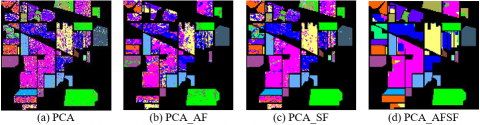Figure 10. The results of Indian Pines scene classification by different methods

## 11.png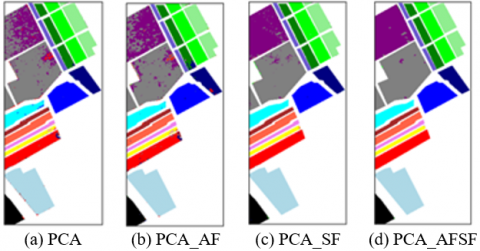Figure 11. The results of University of Pavia scene classification by different methods

Table 4. The precision statistical of University of Pavia scene

 Classification Method PCA PCA_AF PCA PCA_AFSF Alfalfa 70.88 98.06 97.96 98.38 Corn-notill 73.34 99.43 99.62 99.73 Corn-mintill 74.78 99.50 99.56 99.68 Corn-mintill 88.45 99.33 99.36 99.66 Grass-pasture 69.22 99.90 99.92 100.00 Grass-trees 91.94 99.56 99.73 99.89 Grass-pasture-mowed 65.03 99.95 99.81 100.00 Hay-windrowed 97.14 99.96 99.99 100.00 Oats 81.17 99.93 99.97 100.00 Soybean-notill 77.04 99.35 99.40 99.74 Soybean-mintill 86.03 99.71 99.77 100.00 Soybean-clean 74.14 96.44 96.43 96.76 Wheat 98.83 97.41 97.30 97.74 Woods 94.75 99.80 99.81 100.00 Buildings-Grass-Trees-Drives 80.69 99.99 99.91 100.00 Stone-Steel-Towers 76.61 95.91 95.77 96.13 OA 82.85 99.32 99.34 99.40 Kappa 80.51 98.76 98.89 99.20

3.3 Contrastive analysis of different algorithms

To demonstrate its performance, our approach was compared with five state-of-the-arts combined spatial-spectral image classification algorithms, namely, 2D CNN, AEAP, MRC-BLS, GPWV , and low-rank and sparse matrix decomposition (LRaSMD) .

(1) Comparison on Indian Pines (Figure 12)

As shown in Table 5, 2D CNN and AEAP failed to achieve the classification accuracy of 90%, and faced clearly wrong classifications. On the contrary, MRC-BLS classified 90% of the targets accurately. GPWV and LRaSMD achieved 100% classification accuracy on alfalfa, and oats. Overall, our algorithm achieved the best classification accuracy on most targets, and its OA and Kappa coefficient were about 97%. It can be seen that our approach not only improves the classification accuracy, but also effectively removes noises.

(2) Comparison on University of Pavia (Figure 13)

As shown in Table 6, the classification accuracies of all six approaches were above 90%. The last four methods had much higher Kappa coefficient and OA than the first two methods. The indices of MRC-BLS and PCA_AFSF were greater than those of 2D CNN and AEAP, indicating that the fusion of spatial information can improve the classification accuracy of ground objects more effectively than pixel-by-pixel classification. In addition, GPWV and LRaSMD classified all painted metal sheets accurately, leading our algorithm by 0.48% in classification accuracy. However, our algorithm led the other methods in overall OA and Kappa coefficients (>98%).

## 12.png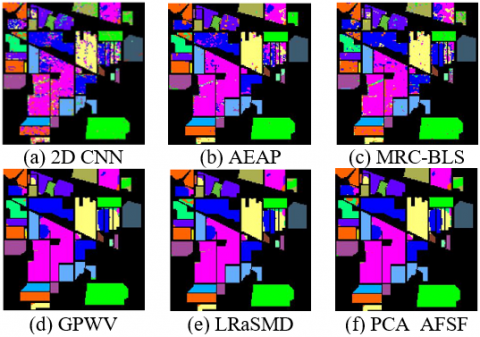Figure 12. The results of Indian Pines scene classification by different methods

## 13.png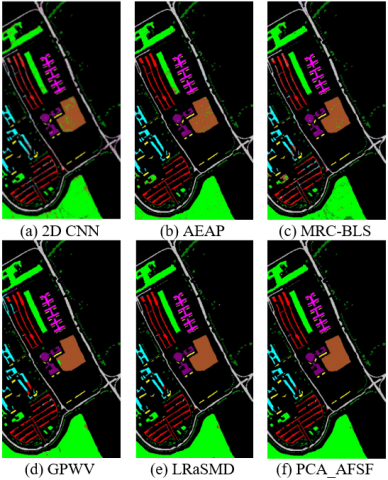Figure 13. The results of University of Pavia scene classification by different methods

Table 5. The precision statistical of Indian Pines scene

 Classification 2D CNN Method AEAP MRC-BLS GPWV LRaSMD PCA_AFSF Alfalfa 94.15 99.99 89.97 100 100 95.86 Corn-notill 88.36 81.95 88.54 94.6 85.95 95.67 Corn-mintill 82.15 77.55 89.96 94.6 94.13 99.82 Corn-mintill 79.95 63.74 99.29 74.4 100 94.12 Grass-pasture 83.93 84.90 98.34 97.8 97.57 99.16 Grass-trees 95.48 95.21 99.59 99.5 99.7 99.96 Grass-pasture-mowed 36.74 60.75 99.04 100 92.86 99.56 Hay-windrowed 98.62 99.14 99.98 100 100 97.05 Oats 76.00 55.40 99.49 100 100 98.74 Soybean-notill 82.80 79.84 87.48 88.1 96.3 99.46 Soybean-mintill 87.83 92.56 83.95 98.3 94.67 97.93 Soybean-clean 85.34 84.40 98.01 94.7 98.66 95.61 Wheat 99.02 98.10 99.69 100 100 99.13 Woods 98.24 97.88 91.09 99.5 98.64 99.21 Buildings-Grass-Trees-Drives 97.17 75.34 98.51 87.5 98.11 99.39 tone-Steel-Towers 97.82 90.37 99.68 90.4 100 97.79 OA 89.16 86.85 91.13 95.41 97.29 97.98 Kappa 87.24 85.67 89.31 94.96 95.32 97.91

Table 6. The precision statistical of University of Pavia scene

 Classification 2D CNN Method AEAP MRC-BLS GPWV LRaSMD PCA_AFSF Asphalt 96.03 93.37 97.30 90.84 94.8 99.21 Meadows 97.38 97.17 98.52 91.90 99.00 99.27 ravel 76.12 87.25 97.62 94.53 90.1 98.68 Trees 86.73 97.91 97.85 96.97 98.81 96.83 Painted metal sheets 97.22 99.43 98.74 100 100 99.52 Bare Soil 77.77 99.30 94.49 91.24 98.50 99.33 Bitumen 66.22 97.16 96.99 100 98.90 99.32 Self-Blocking Bricks 85.72 93.64 93.51 96.12 94.71 97.80 Shadows 99.41 99.34 97.76 100 99.20 93.94 OA 90.61 93.21 97.22 95.28 97.54 98.87 Kappa 87.19 92.97 96.79 95.60 97.12 98.83

3.4 Contrastive analysis of sample size

Our approach intends to achieve ideal classification results on a small training set. Therefore, it is necessary to analyze the influence of sample size on algorithm performance. On Indian Pines, the approaches with and without secondary classification were tested. Both approaches are hyperspectral image classification methods that combine spatial information with spectral information. The only difference lies in whether secondary filtering is implemented using the guided filter. Figure 14 shows the classification accuracy of each approach, as the proportion of training samples expands from 1% to 12%. When the training samples take up less than 9% of all samples, the approach without secondary classification was far less accurate than our algorithm. When the proportion was 7%, the approach without secondary classification was merely 84%, while our approach achieved a classification accuracy of 89%, with an edge of 5%. When the proportion of training samples surpassed 10%, both approaches can accurately classify more than 95% of samples. Thus, our approach can adapt to the insufficient training samples in some scenes.

Finally, the time efficiency of our algorithm was tested by comparing the approaches with and without secondary classification in the following environment: Intel Core i7-8565U CPU @1.80GHz, 8GB RAM; C++; OpenCV functions. The model training time was not counted. The total time of spatial feature extraction and model classification was calculated. On Indian Pines, the approaches with and without secondary classification consumed 2.6s and 3.1s, respectively. Both approaches are established on the SVM. Compared with the approach without secondary classification, our algorithm consumes a similar time, which is mainly spent on the adaptive guided filtering. Hence, different algorithms can be adopted to meet different demands.

## 14.pngFigure 14. Comparison results of Indian Pines scene under different training samples

4. Conclusions

Based on adaptive guided filtering, this paper proposes a combined spatial-spectral hyperspectral image classification approach. By fusing multiple features, a coarse-to-fine framework was established for combined spatial-spectral hyperspectral image classification. The time complexity was reduced through the PCA. In addition, an improved LBP descriptor was designed to illustrate image contrast more accurately. Then, a guided filter was presented based on the adaptive regularization factor. The filter further enhances classification accuracy and reduces noise interference by preventing excessive or insufficient smoothing of target contours in the classification results. Experimental results show that our approach can achieve a high classification accuracy in the presence of a small training set.

Acknowledgments

This work was supported in part by the National Natural Science Foundation of China (Grant No.: 62105328).

References

 Vapnik, V. (1999). The Nature of Statistical Learning Theory. Springer Science & Business Media.

 Marpu, P.R., Pedergnana, M., Dalla Mura, M., Benediktsson, J.A., Bruzzone, L. (2012). Automatic generation of standard deviation attribute profiles for spectral–spatial classification of remote sensing data. IEEE Geoscience and Remote Sensing Letters, 10(2): 293-297. https://doi.org/10.1109/LGRS.2012.2203784

 Benediktsson, J.A., Palmason, J.A., Sveinsson, J.R. (2005). Classification of hyperspectral data from urban areas based on extended morphological profiles. IEEE Transactions on Geoscience and Remote Sensing, 43(3): 480-491. https://doi.org/10.1109/TGRS.2004.842478

 Du, P., Tan, K., Xing, X. (2010). Wavelet SVM in reproducing kernel Hilbert space for hyperspectral remote sensing image classification. Optics Communications, 283(24): 4978-4984. https://doi.org/10.1016/j.optcom.2010.08.009

 Moser, G., Serpico, S.B., Benediktsson, J.A. (2013). Land-cover mapping by Markov modeling of spatial-contextual information in very-high-resolution remote sensing images. Proceeding of the IEEE, 2013, 101(3): 631-651. https://doi.org/10.1109/JPROC.2012.2211551

 Pesaresi, M., Benediktsson, J.A. (2001). A new approach for the morphological segmentation of high-resolution satellite imagery. IEEE Transactions on Geoscience and Remote Sensing, 39(2): 309-320. https://doi.org/10.1109/36.905239

 Myint, S.W., Lam, N.S.N., Tyler, J.M. (2004). Wavelets for urban spatial feature discrimination. Photogrammetric Engineering & Remote Sensing, 70(7): 803-812. https://doi.org/10.14358/pers.70.7.803

 Li, S., Kang, X., Hu, J. (2013). Image fusion with guided filtering. IEEE Transactions on Image Processing, 22(7): 2864-2875. https://doi.org/10.1109/TIP.2013.2244222

 Kang, X., Li, S., Benediktsson, J.A. (2013). Spectral–spatial hyperspectral image classification with edge-preserving filtering. IEEE Transactions on Geoscience and Remote Sensing, 52(5), 2666-2677. https://doi.org/10.1109/TGRS.2013.2264508

 Liu, H., Li, W., Xia, X.G., Zhang, M., Tao, R. (2021). Superpixelwise collaborative-representation graph embedding for unsupervised dimension reduction in hyperspectral imagery. IEEE Journal of Selected Topics in Applied Earth Observations and Remote Sensing, 14: 4684-4698. https://doi.org/10.1109/JSTARS.2021.3077460

 Kang, X., Li, S., Benediktsson, J.A. (2013). Feature extraction of hyperspectral images with image fusion and recursive filtering. IEEE Transactions on Geoscience and Remote Sensing, 52(6): 3742-3752. https://doi.org/10.1109/TGRS.2013.2275613

 Li, R., Cui, K., Chan, R.H., Plemmons, R.J. (2022). Classification of hyperspectral images using SVM with shape-adaptive reconstruction and smoothed total variation. arXiv preprint arXiv:2203.15619. https://doi.org/10.48550/arXiv.2203.15619

 Bhatti, U.A., Yu, Z., Chanussot, J., et al. (2021). Local similarity-based spatial–spectral fusion hyperspectral image classification with deep CNN and Gabor filtering. IEEE Transactions on Geoscience and Remote Sensing, 60: 5514215. https://doi.org/10.1109/TGRS.2021.3090410

 Chen, Y., Jiang, H., Li, C., Jia, X., Ghamisi, P. (2016). Deep feature extraction and classification of hyperspectral images based on convolutional neural networks. IEEE Transactions on Geoscience and Remote Sensing, 54(10): 6232-6251. https://doi.org/10.1109/TGRS.2016.2584107

 Girshick, R., Donahue, J., Darrell, T., Malik, J. (2014). Rich feature hierarchies for accurate object detection and semantic segmentation. 2014 IEEE Conference on Computer Vision and Pattern Recognition, Columbus, OH, USA, pp. 580-587. https://doi.org/10.1109/cvpr.2014.81

 Girshick, R., Donahue, J., Darrell, T., Malik, J. (2015). Region-based convolutional networks for accurate object detection and segmentation. IEEE Transactions on Pattern Analysis and Machine Intelligence, 38(1): 142-158. https://doi.org/10.1109/TPAMI.2015.2437384

 Liu, J., Yang, Z., Liu, Y., Mu, C. (2021). Hyperspectral remote sensing images deep feature extraction based on mixed feature and convolutional neural networks. Remote Sensing, 13(13): 2599. https://doi.org/10.3390/rs13132599

 Karpathy, A., Toderici, G., Shetty, S., Leung, T., Sukthankar, R., Li, F.F. (2014). Large-scale video classification with convolutional neural networks. 2014 IEEE Conference on Computer Vision and Pattern Recognition, Columbus, OH, USA, pp. 1725-1732. https://doi.org/10.1109/CVPR.2014.223

 Haut, J.M., Paoletti, M.E., Plaza, J., Plaza, A., Li, J. (2019). Hyperspectral image classification using random occlusion data augmentation. IEEE Geoscience and Remote Sensing Letters, 16(11): 1751-1755. https://doi.org/10.1109/LGRS.2019.2909495

 Wang, H., Cheng, Y., Chen, C.P., Wang, X. (2021). Semisupervised classification of hyperspectral image based on graph convolutional broad network. IEEE Journal of Selected Topics in Applied Earth Observations and Remote Sensing, 14: 2995-3005. https://doi.org/10.1109/JSTARS.2021.3062642

 Hong, D., Gao, L., Yao, J., Zhang, B., Plaza, A., Chanussot, J. (2020). Graph convolutional networks for hyperspectral image classification. IEEE Transactions on Geoscience and Remote Sensing, 59(7): 5966-5978. https://doi.org/10.1109/TGRS.2020.3015157

 Ma, Y., Liu, Z., Chen, C.P. (2021). Multiscale Random Convolution Broad Learning System for Hyperspectral Image Classification. IEEE Geoscience and Remote Sensing Letters, 19: 5503605. https://doi.org/10.1109/LGRS.2021.3060876

 Zhou, C., Tu, B., Ren, Q., Chen, S. (2021). Spatial peak-aware collaborative representation for hyperspectral imagery classification. IEEE Geoscience and Remote Sensing Letters, 19: 5506805. https://doi.org/10.1109/LGRS.2021.3083416

 Bau, T.C., Sarkar, S., Healey, G. (2010). Hyperspectral region classification using a three-dimensional Gabor filterbank. IEEE Transactions on Geoscience and Remote Sensing, 48(9): 3457-3464. https://doi.org/10.1109/TGRS.2010.2046494

 Tang, Y.Y., Lu, Y., Yuan, H. (2014). Hyperspectral image classification based on three-dimensional scattering wavelet transform. IEEE Transactions on Geoscience and Remote Sensing, 53(5): 2467-2480. https://doi.org/10.1109/TGRS.2014.2360672

 Nie, T., Han, X., He, B., Li, X., Liu, H., Bi, G. (2020). Ship detection in panchromatic optical remote sensing images based on visual saliency and multi-dimensional feature description. Remote Sensing, 12(1): 152. https://doi.org/10.3390/rs12010152

 Yin, B., Cui, B. (2021). Multi-feature extraction method based on Gaussian pyramid and weighted voting for hyperspectral image classification. 2021 IEEE International Conference on Consumer Electronics and Computer Engineering (ICCECE), Guangzhou, China, pp. 645-648. https://doi.org/10.1109/ICCECE51280.2021.9342473

 Cao, H., Shang, X., Yu, C., Song, M., Chang, C.I. (2020). Hyperspectral classification using low rank and sparsity matrices decomposition. IGARSS 2020-2020 IEEE International Geoscience and Remote Sensing Symposium, Waikoloa, HI, USA, pp. 477-480. https://doi.org/10.1109/IGARSS39084.2020.9324009